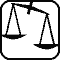Mathematical and Physical Journal
for High Schools
Issued by the MATFUND Foundation
 Already signed up? New to KöMaL?

# Exercises and problems in PhysicsDecember 2004## Experimental problem

M. 256. Take photographs of a working sparkler with sufficiently short exposure times, and estimate the speed of the flakes shooting out with their help. (Be careful when carrying out the experiment!)

(6 points)## Theoretical problems

It is allowed to send solutions for any number of problems, but final scores of students of grades 9-12 are computed from the 5 best score in each month. Final scores of students of grades 1-8 are computed from the 3 best scores in each month.

(3 points)

P. 3753. Two schoolboys wearing ice-skates are standing facing each other. The 60 kg boy pushes his 40 kg mate so that the other picks up a velocity of 3 m/s. How much work is done by them? How far are they from each other in 1.5 seconds? (Neglect friction and air drag.)

(4 points)

P. 3754. When is the initial rate of volume growth of 3 oC water higher, when it is cooled down to 0 oC or when it is warmed up to 6 oC?

(4 points)

P. 3755. We are taking a photograph of the side of the front wheel of a bicycle passing in front of us. Because of the finite exposure time the spokes look blurred. But there are sharp points on the image. Where are they?

(5 points)

P. 3756. A vertical water jet impacts on a solid horizontal plane with a velocity of 2 m/s. What is the velocity of the water spreading over the plane?

(4 points)

P. 3757. Two positive and two negative point charges are placed in the corners of a square insulator frame. All the charges are Q, and the sides of the frame are hinged together. a) How much work is needed to deform the frame into a 60o rhombus? b) How does the magnitude of the electric field change in the center of the frame?

(4 points)

P. 3758. Increasing n times the resistance R connected to a battery the power on the resistor surprisingly does not change. What is the internal resistance of the battery?

(4 points)

P. 3759. An aluminium and a copper wire of the same length and cross-section are connected to a battery in parallel. Which of them begins to warm up faster? How much faster does it warm up?

(4 points)

P. 3760. We connect a resistance of 200 $\displaystyle \Omega$ and a coil with an ohmic resistance of 4 $\displaystyle \Omega$ in series to an electric network of 230 V and 50 Hz. In consequence we can measure 150 V of effective voltage on the coil. a) How much is the inductivity of the coil? b) How much power is absorbed by the coil and by the 200 $\displaystyle \Omega$ resistance?

(4 points)

P. 3761. Where is the electric field strength greatest in a gold atom and how much is it?

(4 points)

P. 3762. A small steel rod due to some pulling force acting on one of its ends is accelerating uniformly from the state of rest in the direction of its longitudinal axis. How many times does the rod cover the distance of its original length when its length measured in the laboratory system is again equal to its original?

(5 points)